# Last 6 Months Questions

You have a stream of integer, implement the following two methods to return the median value (value of the middle. It’s not the mean). The median value does not need to be exact. It can be within a range of 2^n-1 to 2^n+1.
For example:
getMedian() can return any value between 4 to 7.

``````We're given a Grid that is initially empty. Now, we want to play a game on it by placing the character 'X'.
Each time, we can place an 'X' at one of the coordinates in the grid (i, j) and we need to return whether the game has ended or not.

The game is considered to be won when there exists an 'X' path from the first column of the grid to the last column.
We need to efficiently play this game now.
For a path to be winning, we need to consider only the elements in the 4 directions from it...i.e. we can't take up the diagonal elements.

E.g.,

X  _  _  _
_  X  X  X
_  X  X  _
_  _  _  _
_  X  _  _
_  _  _  _

In the above grid, we can see that there is a diagonal path but diagonal paths aren't considered valid and the game isn't ended at that point of time. In the above grid itself, if we now place a new 'X' at (0, 1) index, we'll have the below grid

X  X  _  _
_  X  X  X
_  X  X  _
_  _  _  _
_  X  _  _
_  _  _  _

And now we can go from the leftmost column to the rightmost column traversing only via X's.```E.g.,```We have a chat application and each transaction has a sender and receiver. Every message has a sender user and a receiver user. for every chat message the registerTransaction method is called. at any moment we need a getMostActiveUser which returns the user with most number of other users he/she is transacting with.

Given a playlist of songs, you have to design a song shuffler.
This song shuffler is not like the normal song shuffler that shuffles the complete playlist at the start and returns a shuffled list, but instead when asked for a next song to be played, returns a random song from the list of songs.
The next random song to be played should satisfy a condition that the song was not played in the last ‘k’ turns.
You have to make sure, that at each call, all the eligible (not played during last k turns) songs have equal probability of being played next.

For example:
if songs = [A, B, C, D], k = 2,
then a possible random sequence of songs can be:

``````playNext: [ A , B , C , D ] ->  return C
playNext: [ A , B , _ , D ] ->  return A
playNext: [ _ , B , _ , D ] ->  return B
playNext: [ _ , _ , C , D ] ->  return C (as C was not played in the last two turns, it has an equal probability with D to be played).``````Constraints :
N,M<=5000
MAX(ai,bi) <=5000

Given a string s which contains only curly braces and questions was what is the minimum number of operations to become it valid expression and return corrected string. There are three operations:
insert { or } in any position
delete { or } in any position
flip any position so { change by } or vice versa.

Given N, K and array A length of N and contains non-negative integers. for example N = 5, K = 2, A = [4, 8, 1, 10, 2]. K is initial work capability which means that you can work K days, when you work i-th day your capability decreases by 1 and you get reward A[i], when you skip day your current capability increases by 1. you start at 0 and the question was what is the maximum total reward you can get.
In this example, the answer is 22 because you work 0 and 1 day, after that your capability will become 0. After that you skipp day 2 and capability becomes 1 and you work day 3 and you will get 10 reward, in total 4+8+10=22 reward and it’s the maximum you can achieve.

You are given a course prerequisites list in the form {(a,b),(c,d)…}. A semester is a unit where you can take one or multiple courses. To take a course in a semester, you must complete all the prerequisite courses of that course. You need to find minimum number of semesters required to complete all the courses of the curriculum.
(a,b) -> course a is prerequisite to take course b.
Example: Given (1,2) (1,5) (5,2) (2,3) (2,4) (4,6) (5,6) the answer is 5./Which means you need can complete the curriculum in 5 semesters.

Given some names and their values. Write a function which takes name as input and outputs full value, also replacing the name in the value if present.

``````AXA = '/leetcode/config'
BYB =  '/%AXA%/interview/corner/file'
LCLS = '/tmp/file/usr/shared/%BYB%'

Input: BYB
Output: '/leetcode/config/interview/corner/file'

Input: LCLS
Output: '/tmp/file/usr/shared/leetcode/config/interview/corner/file'``````

Given a Doubly Linked list, find a minimum number of API calls ( move()) to sort the given Linked List. we can only use the move() method to move the node value. We can iterate over the LinkedList elements as many times as we want but the task is to minimize the move() calls and sort the LinkedList.

Example LinkedList 5 -> 2 -> 4 -> 3 -> 1 -> 6

move() – function takes node_val that you want to move and the position of where you want to place the node at. For example, if you want to pick node_val 5 and place it between 1 and 6 you can use move(5,1,6). The interviewer mentioned the move function is flexible and we can use an index as well to move the node. The way the function works is It would delete the node_val 5 and place it between 1 and 6 like this 2->4->3->1->5->6. This is defined as a 1 move() call.

Our task is to minimize the move() call to sort the given LinkedList. For this linkedList 5 -> 2 -> 4 -> 3 -> 1 -> 6, minimum move() calll is 3 to sort the list.

2->4->3->1->5->6 <– move 1
1->2->4->3->5->6 <– move 2
1->2->3->4->5->6 <– move 3Two arrays are given of length `n` and two numbers `c` and `d`.
Find the count of all pairs that follow the condition : `a[i]-a[j]+c <= b[i]-b[j]+d` such that `i < j`.

Constraints:

``````2 <= n < 1e5
1 <= a[i], b[i] <= 1e9``````

You are given the following:

• Integers `n``c``d`
• Array a as `{a1, a2, ..., an}` of length `n`
• Array b as `{b1, b2, ..., bn}` of length `n`

Determine the number of pairs `i``j` (`1 <= i < j <= n`), satisfying the inequality
`ai - aj + c <= bi - bj + d`

Constraints
`1 <= T <= 10`
`2 <= n <= 2.10^5`
`0 <= c, d <= 100`
`1 <= ai, bi <= 10^9`

You are given a string S of lenght N of digits 0 – 9. You need to partiton strings into K substrings such that

• Each substring has a minimum lenght of M
• Substring must start with even digit and ends with odd digit number

Determine the number of ways to partitioin the strings which satisfy the above condition
You should find answer modulo 1e9 + 7

constraints :
1 <= n<= 2×10^3
1<= m<= n
1<=k<=n

Test cases

``````n = 9
m= 2
k = 3
s = '232387421'
So there are total 3 ways possible ``````

You are given an `n x n` integer matrix `grid` where each value `grid[i][j]` represents the elevation at that point `(i, j)`.

The rain starts to fall. At time `t`, the depth of the water everywhere is `t`. You can swim from a square to another 4-directionally adjacent square if and only if the elevation of both squares individually are at most `t`. You can swim infinite distances in zero time. Of course, you must stay within the boundaries of the grid during your swim.

Return the least time until you can reach the bottom right square `(n - 1, n - 1)` if you start at the top left square `(0, 0)`.

Example 1: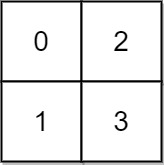```Input: grid = [[0,2],[1,3]]
Output: 3
Explanation:
At time 0, you are in grid location (0, 0).
You cannot go anywhere else because 4-directionally adjacent neighbors have a higher elevation than t = 0.
You cannot reach point (1, 1) until time 3.
When the depth of water is 3, we can swim anywhere inside the grid.
```

Example 2: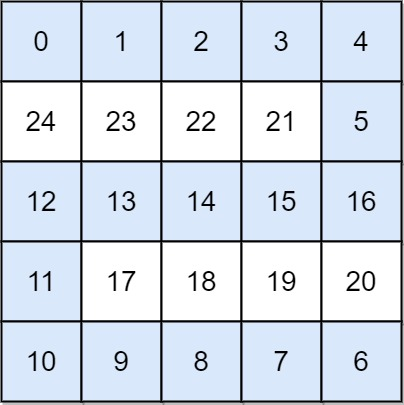```Input: grid = [[0,1,2,3,4],[24,23,22,21,5],[12,13,14,15,16],[11,17,18,19,20],[10,9,8,7,6]]
Output: 16
Explanation: The final route is shown.
We need to wait until time 16 so that (0, 0) and (4, 4) are connected.
```

Constraints:

• `n == grid.length`
• `n == grid[i].length`
• `1 <= n <= 50`
• `0 <= grid[i][j] < n2`
• Each value `grid[i][j]` is unique.

Follow Up – Can Move in All 4 directions

Given an input string, return an output string such that all the lower case characters should be sorted. Indices of non-lower case characters should remain the same as in the original input string.

Output -> ‘Teeg@123 Gloost’

Given a matrix of size n*m, where each position is representing a city.
Initially all city are represented by zero. ( means they are not traversible ).
On each day one city will randomly become traversible. ( matrix[i][j] = 1 )

Write an algorithm that can detect when there is a path from any city of first column to any city of last column.

Given a string s, return the length of longest substring such that every character in the substring has equal number of occurance.

Suppose you are working on Google Photos. you are wring the client application. Request comes to you to upload N photos. you fire the request to server to upload those N photos to server. Then the server responds back with acknowledgements that a particular photo is uploaded.
Example. Suppose you are uploading 10 Photos, The server can respond back in any order, such as 1,2,4,5,3,7,6,10,9,8 . Now at any given point of time we need to check what is the maximum photo number which has been uploaded continously.
Example.

ack(1),
getMax()-> returns 1, because the maximum photo uploaded is 1
ack(2),
getMax()-> returns 2, because the maximum photo uploaded is 2
ack(4)
getMax()-> returns 2 only because 3 has not been recieved yet
ack(5)
getMax()-> returns 2 again because 3 has not been recieved yet
ack(3)
getMax()-> returns 5 because we recieved 3 and 4 and 5 also we recieved eralier. using this example you have to complete the following class

``````public class PhotosClient {

// initializer
public PhotosClient(int n) {

}
// this method is called each time you receive acknowledgement forom the server
public void ack(int x) {

}
// this method will be called in between to check what the maximum photo number has been uploaded successfully
public int getMax() {

}
}``````

Given a horizontal line, you have to paint coordinates and tell the amount of paint required at every step.
Ex – [(4, 10), (7, 13), (20, 30)]
ans – [6, 3, 10]

Given an integer array `a`, and 2 integers – `c` and `k`, where `c` is the favourite number and `k` is the maximum number of replacements we can make. We have to find the maximum number of contiguous elements which are equal to `c`. We can replace `k` number of elements with any number we want.

Example – `a = [1,2,2,3,2,2,2,4], c=2, k=2`.

• In this case, without any replacement, maximum number of contiguous elements will be 3 (from index `4` to index `6`).
• We can replace element at index `3` with number `2` so now array will be `a = [1,2,2,2,2,2,2,4]` , maximum number of contiguous elements will be 6 (from index `1` to index `6`).
• For last replacement, we can replace either element at index `0` or index `7` with number `2`, so maximum number of contiguous elements will be `7`, which is the answer.

You have boxes in magazine and a single empty spot (at the end) like:

1, 4, 2, 5, 3, _

You have a robot which needs to sort boxes. It can only pick up single box, put it in empty box and then pick another one up. Sort boxes to have either _, 1, 2, 3, 4, 5 or 1, 2, 3, 4, 5, _
What is the fastest way to sort if robot knows immediately where are boxes with specific numbers, but it takes time for him to move.

Evaluate the value of an arithmetic expression in Reverse Polish Notation.

Valid operators are `+``-``*`, and `/`. Each operand may be an integer or another expression.

Note that division between two integers should truncate toward zero.

It is guaranteed that the given RPN expression is always valid. That means the expression would always evaluate to a result, and there will not be any division by zero operation.

Example 1:

```Input: tokens = ["2","1","+","3","*"]
Output: 9
Explanation: ((2 + 1) * 3) = 9
```

Example 2:

```Input: tokens = ["4","13","5","/","+"]
Output: 6
Explanation: (4 + (13 / 5)) = 6
```

Example 3:

```Input: tokens = ["10","6","9","3","+","-11","*","/","*","17","+","5","+"]
Output: 22
Explanation: ((10 * (6 / ((9 + 3) * -11))) + 17) + 5
= ((10 * (6 / (12 * -11))) + 17) + 5
= ((10 * (6 / -132)) + 17) + 5
= ((10 * 0) + 17) + 5
= (0 + 17) + 5
= 17 + 5
= 22
```

Constraints:

• `1 <= tokens.length <= 104`
• `tokens[i]` is either an operator: `"+"``"-"``"*"`, or `"/"`, or an integer in the range `[-200, 200]`.

You are a hiker preparing for an upcoming hike. You are given `heights`, a 2D array of size `rows x columns`, where `heights[row][col]` represents the height of cell `(row, col)`. You are situated in the top-left cell, `(0, 0)`, and you hope to travel to the bottom-right cell, `(rows-1, columns-1)` (i.e., 0-indexed). You can move updownleft, or right, and you wish to find a route that requires the minimum effort.

A route’s effort is the maximum absolute difference in heights between two consecutive cells of the route.

Return the minimum effort required to travel from the top-left cell to the bottom-right cell.

Example 1: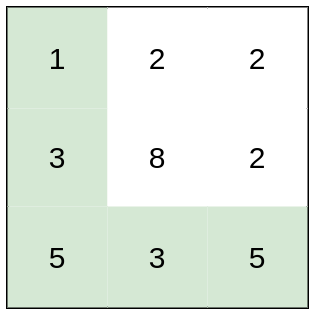```Input: heights = [[1,2,2],[3,8,2],[5,3,5]]
Output: 2
Explanation: The route of [1,3,5,3,5] has a maximum absolute difference of 2 in consecutive cells.
This is better than the route of [1,2,2,2,5], where the maximum absolute difference is 3.
```

Example 2: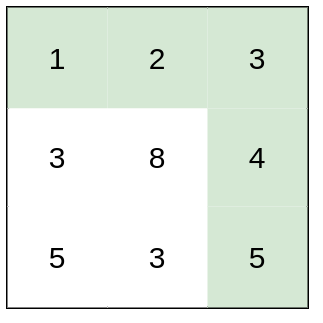```Input: heights = [[1,2,3],[3,8,4],[5,3,5]]
Output: 1
Explanation: The route of [1,2,3,4,5] has a maximum absolute difference of 1 in consecutive cells, which is better than route [1,3,5,3,5].
```

Example 3: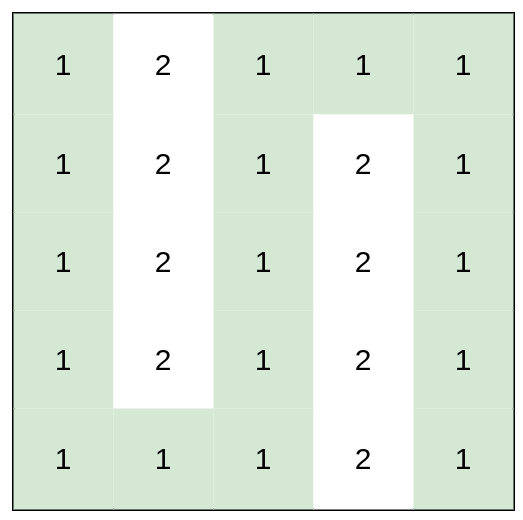```Input: heights = [[1,2,1,1,1],[1,2,1,2,1],[1,2,1,2,1],[1,2,1,2,1],[1,1,1,2,1]]
Output: 0
Explanation: This route does not require any effort.
```

Constraints:

• `rows == heights.length`
• `columns == heights[i].length`
• `1 <= rows, columns <= 100`
• `1 <= heights[i][j] <= 106`

Given a `m x n` matrix `mat` and an integer `threshold`, return the maximum side-length of a square with a sum less than or equal to `threshold` or return `0` if there is no such square.

Example 1: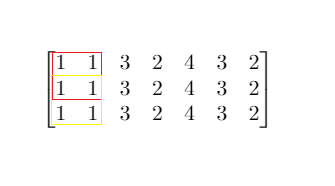```Input: mat = [[1,1,3,2,4,3,2],[1,1,3,2,4,3,2],[1,1,3,2,4,3,2]], threshold = 4
Output: 2
Explanation: The maximum side length of square with sum less than 4 is 2 as shown.
```

Example 2:

```Input: mat = [[2,2,2,2,2],[2,2,2,2,2],[2,2,2,2,2],[2,2,2,2,2],[2,2,2,2,2]], threshold = 1
Output: 0
```

Constraints:

• `m == mat.length`
• `n == mat[i].length`
• `1 <= m, n <= 300`
• `0 <= mat[i][j] <= 104`
• `0 <= threshold <= 105`

To install packages of different versions of an Android app, each package has different Android system requirements (only the major version is considered). For example:
v1: min 1.0 max 6.0
v2: min 3.0 max 7.0
v3: min 4.0 max 9.0

Find the Android system from 0.0 -> latest (the interviewer said it is MAX_INTEGER) different Android installation packages supported by each interval, and then only return all intervals:
[0.0, 0.0] [1.0,2.0] [3.0, 6.0] [7.0, 7.0] [8.0, latest] -> return these intervals
none v1 v1,v2 v2 none -> do not need to return

U need to design structure in which it will nee to implement following 2 methods :

1. public void insertOrReplace(long index, long number)
2. public long findSmallestIndex(long number)
so the first one is to insert the number on the index given by the user, index can be any number of long type and if at the same index another number comes it will replace that number
2nd method is need to be implemented int which user will be given any number and we need to return the smallest index of that number
Ex :
index->number sequence
2->100
1->100
3->100
5->100
if any user does findSmallestIndex(100) , output will be 1
2-> 200
if new number 200 comes at index 2 then 100 will be present only at indexs 1,3 &5 and 2nd index will be removed.

Given T[] , an array that represents N agents and the time they need to serve customers . All N agents can serve at oce
for M customers if they are waiting , they choose the agent with least serving time . If a New customer comes in , calculate the total wait time.

1. what if client number is far greater than agent number?
2. what if there’s a constraint on time[i] that 1 <= time[i] <= 10, how would you use this to your advantage?

8 directions: N\W\E\S\NW\NE\SW\SE
check whether the input is valid?
input : P1NP2,P2NP3,P3NP1

P1 is North of P2
P2 is North of P3
P3 is North of P1
Output: False (since P3 will be in the South of P1)

A board (int[][] matrix) only has 0 and 1. Each time call next() to add a 1 at matrix, and the other numbers shift (in Z path)…if any col or row are all 1s, return true. or false.
example :
101
010
111
after call next():
110
101
011
after call next():
111
010
101

Graph is given as adj list format, return nodes order starting from any node.
Input:
[A: [B, D]
B: [A, C]
C: [B, D]
D: [A, C]]

Output: ABCD

Assumption: Graph represents Ring topology find the optimized way to traverse.
Follow up: Check if Graph represent ring topology check for all corner cases.

Design a game 5×5. Given getRandom(start, end) function to generate all random numbers from 1 to 75

[[1, 5, 2, 8, 13] number random from 1 to 15
[19,17,16,25] number random from 16 to 30
[35,32,43,41,39] 31 to 45
[etc…]

Create a void function which generate the game and return 2D array which contains game

no input (void function)
output will be 2D array

Give a string, for example : input : a-(b+c+b) output : a-2b-c.

Remove Leaves of Binary Tree , like (366. Find Leaves of Binary Tree) , but need remove

Follow up 1 – Remove new created leaves first (after remove 4,5, we need remove 2 immediately..then 6,7 ….)
1
/ \
2 3
/ \ / \
4 5 6 7

Follow up 2 – Remove new created leaves last

A binary tree, return all root-to-node paths. For example,
1
/ \
2 3
\
5
return : [“1″,”12″,”125″,”13”]

Given an index and an input string find the char at the given index of the string. But here’s the catch: if the index exceeds the length of the string, then you transform the string by removing the string’s last character and appending it to the front and appending this transformed string to the original string until you have a string length that exceeds the desired index.

For example,if we have a string “abcd” and want to find the char at index 3 it would obviously be ‘d’. However, if we want to find the char at index 7 of “abcd” we would transform “abcd” to “abcddabc” and the char would be ‘c’. If we want to find the char at index 12 of “abcd” we would transform “abcd” to “abcddabc” and then again transform to “abcddabccabcddab” and the char would be ‘d.

path in a binary tree is a sequence of nodes where each pair of adjacent nodes in the sequence has an edge connecting them. A node can only appear in the sequence at most once. Note that the path does not need to pass through the root.

The path sum of a path is the sum of the node’s values in the path.

Given the `root` of a binary tree, return the maximum path sum of any non-empty path.

Example 1: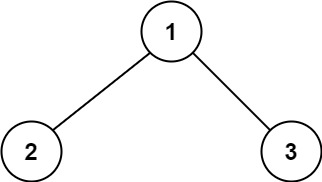```Input: root = [1,2,3]
Output: 6
Explanation: The optimal path is 2 -> 1 -> 3 with a path sum of 2 + 1 + 3 = 6.
```

Example 2: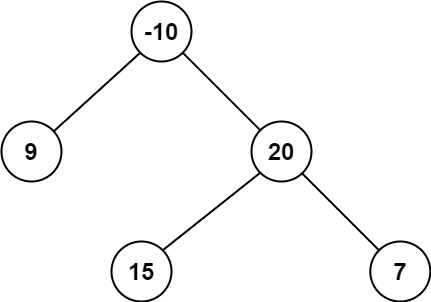```Input: root = [-10,9,20,null,null,15,7]
Output: 42
Explanation: The optimal path is 15 -> 20 -> 7 with a path sum of 15 + 20 + 7 = 42.
```

Constraints:

• The number of nodes in the tree is in the range `[1, 3 * 104]`.
• `-1000 <= Node.val <= 1000`

Follow up – Find the Maximum Path sum of the path with the exact length of K.

Given a sorted integer array `arr`, two integers `k` and `x`, return the `k` closest integers to `x` in the array. The result should also be sorted in ascending order.

An integer `a` is closer to `x` than an integer `b` if:

• `|a - x| < |b - x|`, or
• `|a - x| == |b - x|` and `a < b`

Example 1:

```Input: arr = [1,2,3,4,5], k = 4, x = 3
Output: [1,2,3,4]
```

Example 2:

```Input: arr = [1,2,3,4,5], k = 4, x = -1
Output: [1,2,3,4]
```

Constraints:

• `1 <= k <= arr.length`
• `1 <= arr.length <= 104`
• `arr` is sorted in ascending order.
• `-104 <= arr[i], x <= 104`

Given two integer arrays `nums1` and `nums2`, return an array of their intersection. Each element in the result must be unique and you may return the result in any order.

Example 1:

```Input: nums1 = [1,2,2,1], nums2 = [2,2]
Output: 
```

Example 2:

```Input: nums1 = [4,9,5], nums2 = [9,4,9,8,4]
Output: [9,4]
Explanation: [4,9] is also accepted.
```

Constraints:

• `1 <= nums1.length, nums2.length <= 1000`
• `0 <= nums1[i], nums2[i] <= 1000`

Given the user and there posts, maximize the likes of N posts where N appears atleast N times.
For eg. Consider likes = [8,6,5,5,2,1] Output: 4 (Because there are 4 post with 4 likes. )
Explaination: 8 contains 4 likes, 6 contains 4 likes,5 contains 4 likes,5 contains 4 likes and more importantly number of posts are 4
2. likes = [1,2,2] Output 2 (Because there are 2 post with 2 likes)
3. likes = [1,3,4,2] Output 2(Because there are 2 post with 2 likes)

``````Given T[] , an array that represents N agents and the time they need to serve customers . All N agents can serve at oce
for M customers if they are waiting , they choose the agent with least serving time . If a New customer comes in , calculate the total wait time.``````
``````Given a table of rules, write a function to check if it contains conflicting rules. Two rules are conflicting if their conditions match but values do not. Conditions can contain don't cares denoted by '-'. Has to be done in O(n) time.

e.g
Rule Conditions Value
R1 C1,C2,C3 40
R2 BCD1,BC5 40
R3 BCD1,BC5 80
Here, R2 and R3 conflict.
e.g
R1 C1,C2,C3 40
R2 BCD1,BC5 50
R3 BCD1,- 50
R6 -,-,C3 40
No conflicts.``````

There are a total of `numCourses` courses you have to take, labeled from `0` to `numCourses - 1`. You are given an array `prerequisites` where `prerequisites[i] = [ai, bi]` indicates that you must take course `bi` first if you want to take course `ai`.

• For example, the pair `[0, 1]`, indicates that to take course `0` you have to first take course `1`.

Return the ordering of courses you should take to finish all courses. If there are many valid answers, return any of them. If it is impossible to finish all courses, return an empty array.

Example 1:

```Input: numCourses = 2, prerequisites = [[1,0]]
Output: [0,1]
Explanation: There are a total of 2 courses to take. To take course 1 you should have finished course 0. So the correct course order is [0,1].
```

Example 2:

```Input: numCourses = 4, prerequisites = [[1,0],[2,0],[3,1],[3,2]]
Output: [0,2,1,3]
Explanation: There are a total of 4 courses to take. To take course 3 you should have finished both courses 1 and 2. Both courses 1 and 2 should be taken after you finished course 0.
So one correct course order is [0,1,2,3]. Another correct ordering is [0,2,1,3].
```

Example 3:

```Input: numCourses = 1, prerequisites = []
Output: 
```

Constraints:

• `1 <= numCourses <= 2000`
• `0 <= prerequisites.length <= numCourses * (numCourses - 1)`
• `prerequisites[i].length == 2`
• `0 <= ai, bi < numCourses`
• `ai != bi`
• All the pairs `[ai, bi]` are distinct.

Implement a function getChar(String s, int i) which returns the ith character in s (0-indexed).
We are interested in a special version which return a character even if i >= s.length()

getChar(“abc”, 1) -> ‘b’
getChar(“abc”, 5) -> ‘b’

The string should transform like below. Basically do the following => ABC + append the same string again, just move the last char to the front
abc -> abc+c+ab -> abccab+b+abcca -> abccabbabcca+a +abccabbabcc

You are given a symbolic mathematical expression:
1+(2/3)
2*x
25.3y+(3a+c);
Design a data structure to implement this and evaluate the expression.
Note: We dont need to write the parsing code for this?

Your car starts at position `0` and speed `+1` on an infinite number line. Your car can go into negative positions. Your car drives automatically according to a sequence of instructions `'A'` (accelerate) and `'R'` (reverse):

• When you get an instruction `'A'`, your car does the following:
• `position += speed`
• `speed *= 2`
• When you get an instruction `'R'`, your car does the following:
• If your speed is positive then `speed = -1`
• otherwise `speed = 1`

For example, after commands `"AAR"`, your car goes to positions `0 --> 1 --> 3 --> 3`, and your speed goes to `1 --> 2 --> 4 --> -1`.

Given a target position `target`, return the length of the shortest sequence of instructions to get there.

Example 1:

```Input: target = 3
Output: 2
Explanation:
The shortest instruction sequence is "AA".
Your position goes from 0 --> 1 --> 3.
```

Example 2:

```Input: target = 6
Output: 5
Explanation:
The shortest instruction sequence is "AAARA".
Your position goes from 0 --> 1 --> 3 --> 7 --> 7 --> 6.
```

Constraints:

• `1 <= target <= 104`

You are given an `m x n` integer matrix `grid` where each cell is either `0` (empty) or `1` (obstacle). You can move up, down, left, or right from and to an empty cell in one step.

Return the minimum number of steps to walk from the upper left corner `(0, 0)` to the lower right corner `(m - 1, n - 1)` given that you can eliminate at most `k` obstacles. If it is not possible to find such walk return `-1`.

Example 1: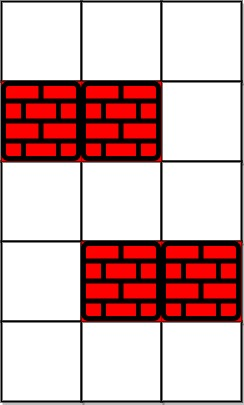```Input: grid = [[0,0,0],[1,1,0],[0,0,0],[0,1,1],[0,0,0]], k = 1
Output: 6
Explanation:
The shortest path without eliminating any obstacle is 10.
The shortest path with one obstacle elimination at position (3,2) is 6. Such path is (0,0) -> (0,1) -> (0,2) -> (1,2) -> (2,2) -> (3,2) -> (4,2).
```

Example 2:```Input: grid = [[0,1,1],[1,1,1],[1,0,0]], k = 1
Output: -1
Explanation: We need to eliminate at least two obstacles to find such a walk.
```

Constraints:

• `m == grid.length`
• `n == grid[i].length`
• `1 <= m, n <= 40`
• `1 <= k <= m * n`
• `grid[i][j]` is either `0` or `1`.
• `grid == grid[m - 1][n - 1] == 0`

You are given an array `points`, an integer `angle`, and your `location`, where `location = [posx, posy]` and `points[i] = [xi, yi]` both denote integral coordinates on the X-Y plane.

Initially, you are facing directly east from your position. You cannot move from your position, but you can rotate. In other words, `posx` and `posy` cannot be changed. Your field of view in degrees is represented by `angle`, determining how wide you can see from any given view direction. Let `d` be the amount in degrees that you rotate counterclockwise. Then, your field of view is the inclusive range of angles `[d - angle/2, d + angle/2]`.

You can see some set of points if, for each point, the angle formed by the point, your position, and the immediate east direction from your position is in your field of view.

There can be multiple points at one coordinate. There may be points at your location, and you can always see these points regardless of your rotation. Points do not obstruct your vision to other points.

Return the maximum number of points you can see.

Example 1: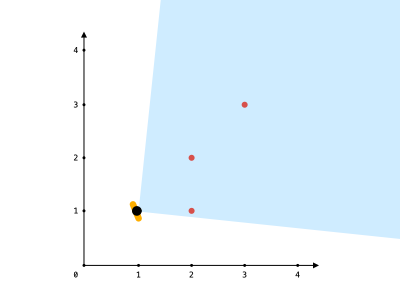```Input: points = [[2,1],[2,2],[3,3]], angle = 90, location = [1,1]
Output: 3
Explanation: The shaded region represents your field of view. All points can be made visible in your field of view, including [3,3] even though [2,2] is in front and in the same line of sight.
```

Example 2:

```Input: points = [[2,1],[2,2],[3,4],[1,1]], angle = 90, location = [1,1]
Output: 4
Explanation: All points can be made visible in your field of view, including the one at your location.
```

Example 3: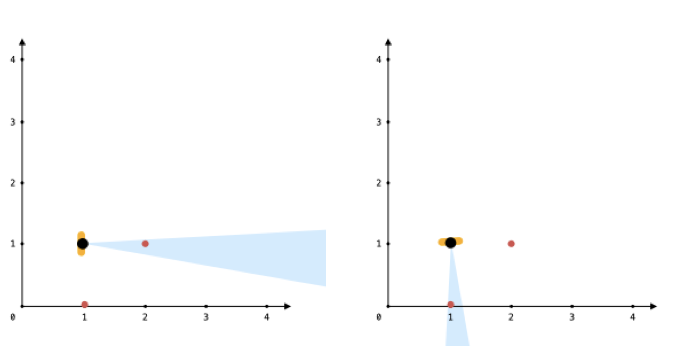```Input: points = [[1,0],[2,1]], angle = 13, location = [1,1]
Output: 1
Explanation: You can only see one of the two points, as shown above.
```

Constraints:

• `1 <= points.length <= 105`
• `points[i].length == 2`
• `location.length == 2`
• `0 <= angle < 360`
• `0 <= posx, posy, xi, yi <= 100`

Design a sparse vector class with below methods

1. void SetValue(int index , int value)
2. int GetValue(int index)
3. int GetNumberOfZeros()

Given a dictionary of words (sorted lexicographically) and a prefix string, return all the words that start with the given prefix.

Follow-up: What if the input is not sorted?

Given a n-ary tree, print the nodes in the required format.
Follow-up: given the printed string, make the tree and return the root.

``````Example:
1
/       |       \
2        3        4
|       /  \      |
5      6    7     8
|
9
print the output in this format (append a dash "-" to each node value and keep adding dashes for each level)
-1
--2
---5
--3
---6
---7
----9
--4
---8``````

There are a total of `numCourses` courses you have to take, labeled from `0` to `numCourses - 1`. You are given an array `prerequisites` where `prerequisites[i] = [ai, bi]` indicates that you must take course `bi` first if you want to take course `ai`.

• For example, the pair `[0, 1]`, indicates that to take course `0` you have to first take course `1`.

Return the ordering of courses you should take to finish all courses. If there are many valid answers, return any of them. If it is impossible to finish all courses, return an empty array.

Example 1:

```Input: numCourses = 2, prerequisites = [[1,0]]
Output: [0,1]
Explanation: There are a total of 2 courses to take. To take course 1 you should have finished course 0. So the correct course order is [0,1].
```

Example 2:

```Input: numCourses = 4, prerequisites = [[1,0],[2,0],[3,1],[3,2]]
Output: [0,2,1,3]
Explanation: There are a total of 4 courses to take. To take course 3 you should have finished both courses 1 and 2. Both courses 1 and 2 should be taken after you finished course 0.
So one correct course order is [0,1,2,3]. Another correct ordering is [0,2,1,3].
```

Example 3:

```Input: numCourses = 1, prerequisites = []
Output: 
```

Constraints:

• `1 <= numCourses <= 2000`
• `0 <= prerequisites.length <= numCourses * (numCourses - 1)`
• `prerequisites[i].length == 2`
• `0 <= ai, bi < numCourses`
• `ai != bi`
• All the pairs `[ai, bi]` are distinct.

Everyone is well aware of Google Maps. We are Installing a new feature into Google Maps. The feature would immediately output the minimum speed required to make a trip.

You are given a sequence of distances between places in the trip. You are given a threshold time. You need to make a trip within this time. Output the minimum speed.

NOTE – [ Time will never be in Fractions/Decimals ]

Example :-

INPUT –
Distance – [5,6,3,9,4]
Threshold Time – 8

OUTPUT –
5

Explanation –
For the Distance – [5,6,3,9,4] with a minimum speed 5, I can cover 5 units distance at 0th index in 1 unit time. Can cover 6 units distance at 1st index in 2 unit time. Can cover 3 units distance at 2nd index in 1 unit time. Can cover 9 units distance at 3rd index in 2 unit time. Can cover 4 units distance at 4th index in 1 unit time.
Total Time Taken – 7 Units which is less than the Threshold of 8 units. So 5 unit speed is the minimum speed possible to make the entire trip.